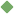newton's_third_law_of_motion 1. [ noun ] (physics,mechanics) action and reaction are equal and opposite Synonyms: Newton's_third_law law_of_action_and_reaction third_law_of_motion Related terms: Newton's_law_of_motion To share this definition press "text" (Facebook, Twitter) or "link" (blog, mail) then paste text link Similar spelling: Newton's_first_law_of_motion   Newton's_second_law_of_motion   Newton's_law_of_gravitation   newton's_theory_of_gravitation